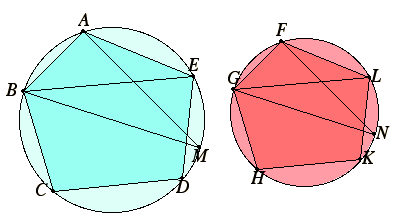# Proposition 1

Similar polygons inscribed in circles are to one another as the squares on their diameters.

Let ABC and FGH be circles, let ABCDE and FGHKL be similar polygons inscribed in them, and let BM and GN be diameters of the circles.

I say that the square on BM is to the square on GN as the polygon ABCDE is to the polygon FGHKL.

Join BE, AM, GL, and FN.VI.Def.1

Now, since the polygon ABCDE is similar to the polygon FGHKL, therefore the angle BAE equals the angle GFL, and BA is to AE as GF is to FL.

VI.6

Thus BAE and GFL are two triangles which have one angle equal to one angle, namely the angle BAE equal to the angle GFL, and the sides about the equal angles proportional, therefore the triangle ABE is equiangular with the triangle FGL. Therefore the angle AEB equals the angle FLG.

III.27

But the angle AEB equals the angle AMB, for they stand on the same circumference, and the angle FLG equals the angle FNG, therefore the angle AMB also equals the angle FNG.

But the right angle BAM also equals the right angle GFN, therefore the remaining angle equals the remaining angle. Therefore the triangle ABM is equiangular with the triangle FGN.

VI.4

Therefore, proportionally BM is to GN as BA is to GF.

VI.20

But the ratio of the square on BM to the square on GN is duplicate of the ratio of BM to GN, and the ratio of the polygon ABCDE to the polygon FGHKL is duplicate of the ratio of BA to GF.

Therefore the square on BM is to the square on GN as the polygon ABCDE is to the polygon FGHKL.

Therefore, similar polygons inscribed in circles are to one another as the squares on their diameters.

Q.E.D.

## Guide

Proposition VI.20 states that the ratio of similar polygons is duplicate the ratio of their corresponding sides, so all that is needed is that the corresponding sides are proportional to the diameters of the circumscribed circles, a result that constitutes the bulk of the straightforward proof.

This proposition is in preparation for the next in which it is shown that circles are proportional to the squares on their diameters. The connection is that the circles can be arbitrarily closely approximated by polygons, so that if the polygons are proportional to the squares, then so will the circles be proportional to the squares. The difficulty in that proof coming up is to make that argument rigorous.